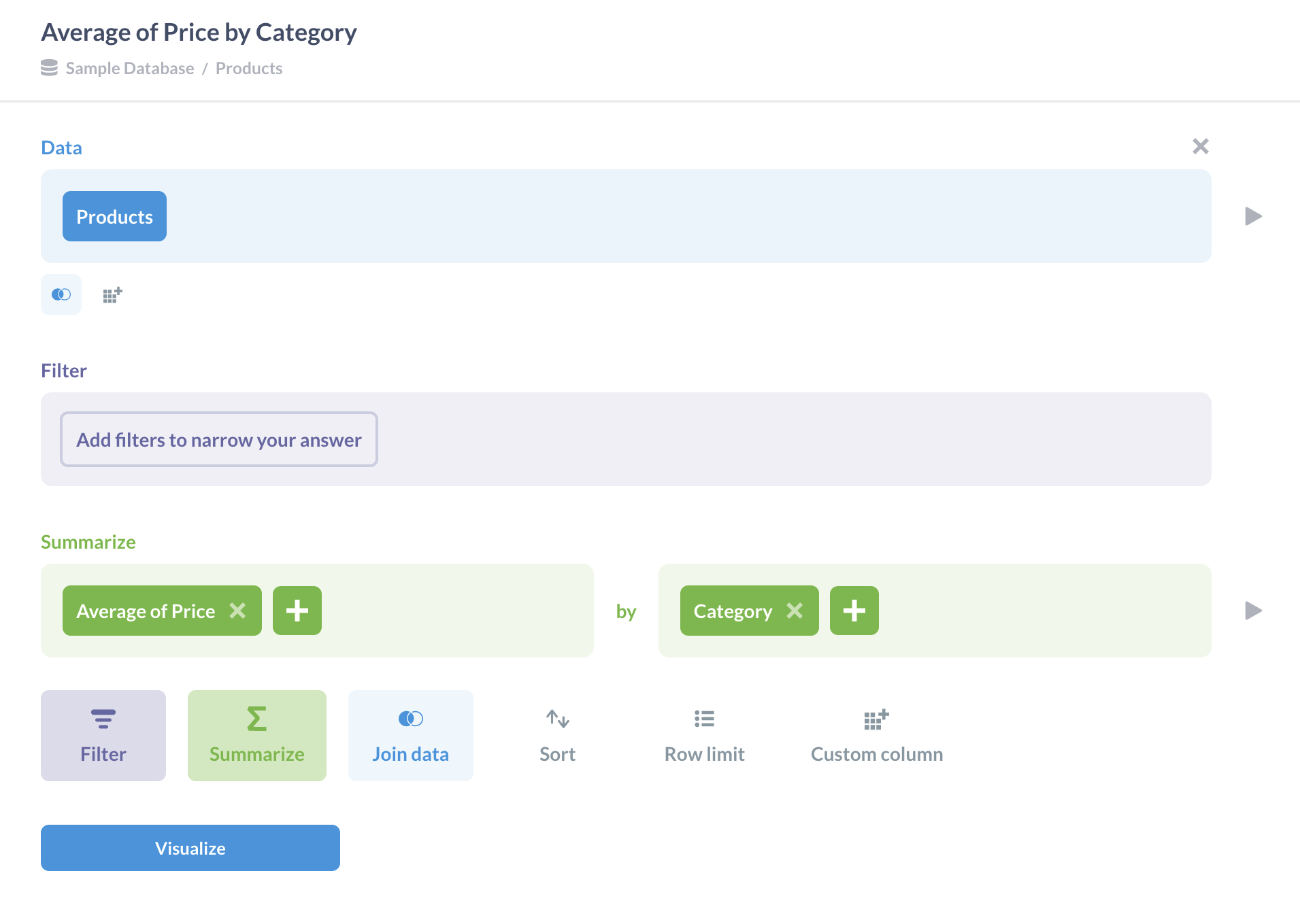# Aggregation

## What is an aggregation?

An aggregation is the act of summarizing data with a mathematical function, such as averaging the values in a column, or counting the number of rows in a table. The resulting number is often called a metric, which is distinct from metrics in Metabase.

Individual values in a field may not hold much meaning on their own, but when we combine these values in some specific way, we can paint a more comprehensive picture of our data. Aggregation is the process of collapsing those values into a single result, and is usually performed in conjunction with grouping — that is, combining multiple rows based on a certain value, like grouping by a dimension (e.g., a product category or country).

Aggregations can be calculated on the fly, but you may also want to create summary tables with your results and save the results of those aggregate functions for future use. Summary tables can be especially useful when working with large datasets; since summary tables are precomputed, queries that rely on them can run much faster.

## Common aggregate functions in SQL

Different databases have different sets of functions, but here are some of the most common aggregate functions that you’ll encounter:

• `COUNT()` - Counts the number of rows in a table.
• `AVG()` – Computes the average of values in a field.
• `MIN()` – Identifies the minimum value in a field.
• `MAX()` – Identifies the maximum value in a field.
• `SUM()` – Returns the sum of values in a field.
• `STDEV()` – Calculates the standard deviation of values in a field.

## Example aggregation

Using Metabase’s Sample Database, let’s say we wanted to know the average price of our products, grouped by product category. In this case, we’ll use the `Products` table. Our SQL query would look like this:

``````SELECT
category,
avg(price)
FROM products
GROUP BY category
``````

Just like we wanted, we’ve calculated the average of values in the `Price` column of our `Products` table, and grouped those averages according to their value in the `Category` field.

If we wanted to execute that same aggregation in Metabase’s query builder, we’d Summarize by Average of `Price`, and then group by `Category`, like in the image below:Fig. 1. Performing an aggregation in the query builder: average price of products grouped by product category.